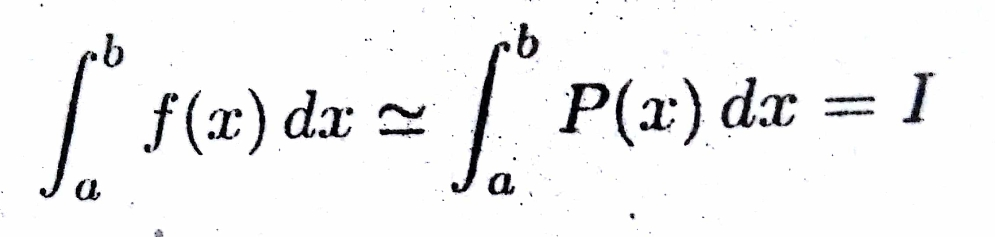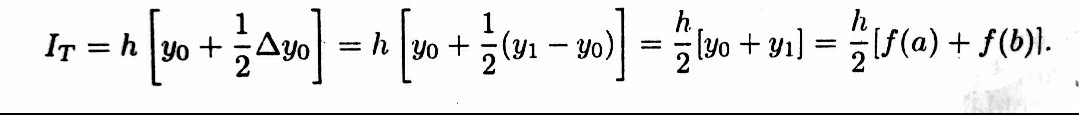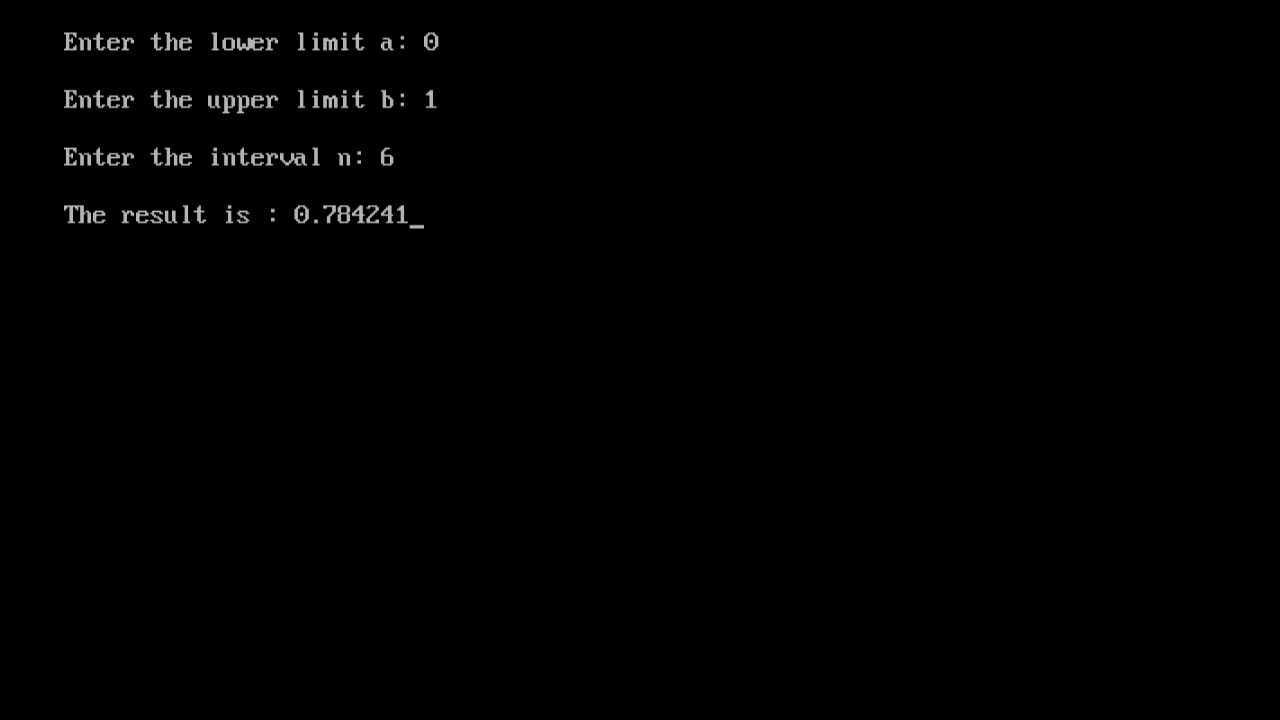# Trapezoidal Rule: Algortihm, Flowchart, Program in CSuppose we are interested to evaluate the definite integralwhen, f(x) is known for a set of (n+1) points or nodes x0,x1,x2,...,xn. Firstly, we have to consider a polynomial P(x) is a degree, which replaces f(x) on the set of n+1 interpolating points xj (j=0,1,2,...,n) i.e., f(xj) = P(xj) (j=0,1,2,...,n). Then we integrate within the limit [a,b] i.e.,and the differenceis called error is approximations. Now for solving such integrals using a two-points quadrature formula is applied and that is the Trapezoidal Rule. There are only two functional values y0=f(x0)=f(a) and y1=f(xn)=f(b), where b-a=h. Thus the second and higher order differences are not possible.The formula, is known as Trapezoidal Rule for Numerical Integration.

## Computation Scheme

If the range of integration [a,b] be divided into n- equal sub-intervals by the points a=x0, x0+h, x+2h, x0+3h, …, x0+(n-1)h, x0+nh=xn=b and the Trapezoidal Rule be applied in each of the intervals [x0,x0+h], [x0+h,x0+2h], …, [x0+(n-2)h,x0+(n-1)h], [x0+(n-1)h,x0+nh], we get,This formula is called Composite Trapezoidal Rule.

## Algorithm and Flowchart:

### Algorithm:

STEP 1: Read N(the number of intervals), A, B(the limits of the integral)
STEP 2: Define F(X)
STEP 3: Compute H=(B-A)/N, the interval size and P=[F(A)+F(B)]/2
STEP 4: Initialize I=1 and Compute the sum P=P+F(A+I*H)
STEP 5: If I=N, then print TRAPZED=H*P, the value of the integral and terminate the process, otherwise replace I by I+1 and Goto STEP 3.

### Flowchart:### Sample Program:

WAP in C to evaluate Integration of f(x) between 0 and 1 using Trapezoidal Rule with 6 intervals, where f(x)=1/(1+x*x)

### Code:

``````#include<stdio.h>
#include<conio.h>
#include<math.h>                /*included the header files*/
void main()
{
float a,b,h,x,y,y0,yn,xn,s,r;
int i,n;
float f(float);         /*calling the function f(x)*/
clrscr();
printf("\nEnter the lower limit a: ");
scanf("%f",&a);
printf("\nEnter the upper limit b: ");
scanf("%f",&b);
printf("\nEnter the interval n: ");
scanf("%d",&n);                   /*Accepting the values of
lower limit, upper limit
and the no of intervals*/
h=(b-a)/n;
y0=f(a);                          /*assigning the values of
functions to variables*/
yn=f(b);
x=a+h;
s=0;
for(i=1;i<n;i++)
{
y=f(x);
s=s+y;
x=x+h;
}
r=(h/2)*((y0+yn)+2*s);         /*applying the trapezoidal formula*/
printf("\nThe result is : %f",r); /*final result printed*/
getch();
}
float f(float x)
{
return (1/(1+pow(x,2)));      /*declaring the function*/
}``````

### Compiler Window:## Output Window:## Video Tutorial:

Feel free to comment below if you are facing problems while executing the program.